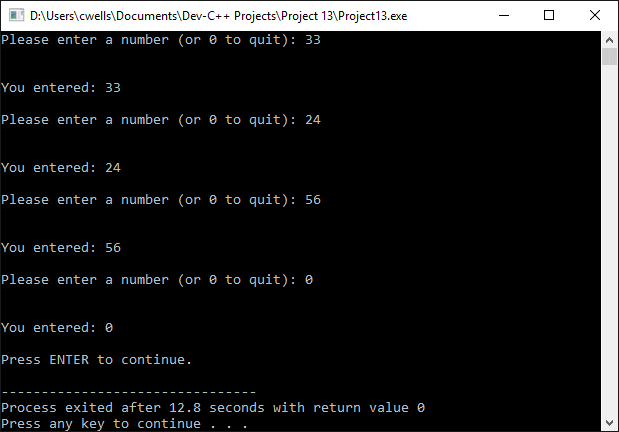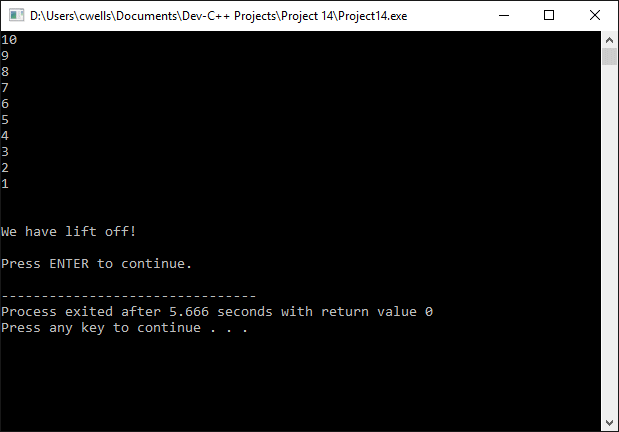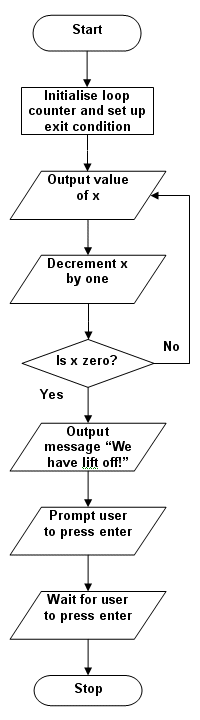# Control Structures

A program's code does not usually execute in a purely linear way. Program execution may require certain program statements, or groups of program statements, to be executed repeatedly (a program loop), either for a predetermined number of iterations, or until some criterion (the exit condition) has been satisfied. Program execution may also branch (jump) to a different location within the program from time to time, depending on (or conditional upon) the current status of one or more variables. Often, the statements that will be executed repeatedly within a loop, or as the result of a conditional statement, are grouped together within a program block. This is a group of program statements, separated by semicolons, but grouped together between curly braces ({ . . . }), as shown below.

{
statement1;
statement2;
statement3;
}

We have actually seen this construct used in many previous example programs to group together all of the program code that makes up the program's main() function. A code block can contain both simple program statements (i.e. single lines of code, each ending in a semi-colon) and compound program statements (i.e. several simple program statements grouped together and enclosed within curly braces). As you will see, the convention when writing code is to indent the code that appears within a code block to make it clearly distinguishable from any program code outside the block. The more deeply nested the block of code, the greater the level of indentation used.

## Conditional structures - if and else

The if keyword is used to execute a statement or a block of code only if a specific condition is met. The general form is shown below.

if (condition) statement

If the expression contained within parentheses evaluates to true, the statement or block of code that follows the condition is executed, otherwise it is not executed. For the following code fragment, the string "x = 25" is only sent to the screen if the value stored in the variable x is 25.

if (x == 25)cout << "x = 25";

If a number of statements are to be executed when a condition evaluates to true, these statements still immediately follow the condition, but they are enclosed between curly braces, as shown below.

if (x == 25)
{
cout << "The value of x is: ";
cout << x;
}

We can also specify an alternative course of action that should be followed if the condition evaluates to false using the keyword else, as shown below.

if (condition) statement1 else statement2

In the example code fragment below, the program outputs the string "x is 50" if the condition is true, otherwise it outputs the string "x is not 50".

if (x == 50)
cout << "x is 50";
else
cout << "x is not 50";

The if . . . else construct can be extended to allow the evaluation of a number of different conditions, with different actions being carried out depending on which of the conditions (if any) evaluates to true. The following code fragment outputs a different string, depending on whether the value of a numeric variable (x) is positive, negative, or neither positive nor negative (i.e. zero).

if (x > 0)
cout << "x is positive";
else if (x < 0)
cout << "x is negative";
else
cout << "x is zero";

## The while loop

A loop construct in a program allows program statements to be executed repeatedly, either for a predetermined number of iterations, or until some criterion (the exit condition been satisfied. One such construct is the while loop, which has the following form:

while (condition) statement;

The statement following the condition (defined within parentheses) is executed repeatedly until the condition evaluates to false. The following short program illustrates the use of the while loop.

// Example Program 1

#include <iostream>
#include <sstream>
using namespace std;

int main ()
{
string s;
int x;

cout << "Please enter a number: ";
getline( cin, s );
stringstream(s) >> x;
while (x > 0)
{
cout << x << ", ";
--x;  // decrement x by 1
}
cout << "\n\n\nPress ENTER to continue.";
getline( cin, s );
return 0;
}The output from example program 1

The program asks the user for a number (x) and prints out a comma-separated list of numbers from x to 0. When the while loop begins, providing the user has entered a number greater than 0, the condition x > 0evaluates to true, and the statements inside the loop will be executed repeatedly until the value of x is no longer greater than 0. The flow chart for the program is shown below.The flow chart for example program 1

## The do-while loop

The do-while loop has the following form:

do statement while (expression);

The do-while loop behaves in exactly the same way as the while loop, with one exception. Whereas the statement in a while loop will only be executed if the condition evaluates to true, the statement in a do-while loop is evaluated at least once, even if the condition evaluates to false on the first iteration of the loop. This is because the condition is evaluated at the end of the loop, instead of at the beginning. The following short program illustrates the use of the do-while loop.

// Example Program 2

#include <iostream>
#include <sstream>
using namespace std;

int main ()
{
string s;
unsigned long x;

do
{
cout << "Please enter a number (or 0 to quit): ";
getline( cin, s );
stringstream(s) >> x;
cout << "\nYou entered: " << x << "\n";
}
while (x != 0);
cout << "Press ENTER to continue.";
getline( cin, s );
return 0;
}The output from example program 2

The loop will continue to accept numbers from the user until the user enters 0, at which point the condition for exiting the loop will have been met. The flow chart for the program is shown below.The flow chart for example program 2

## The for loop

The for loop is essentially a counting loop, because it executes a statement, or block of statements, a pre-determined number of times. The general form of the for loop is as follows:

for (expression1; expression2; expression3) statement;

In this construct, expression1 sets a counter variable to some initial value (this occurs only once). The exit condition is given by expression2, which determines the value the counter must reach in order for program execution to exit the loop. The exit condition is evaluated on each iteration of the loop. During each iteration of the loop, the statements within the loop are executed, as is expression3, which increments (or decrements) the counter by a specified value (usually 1). The following short program illustrates the use of the for loop.

// Example Program 3

#include <iostream>
using namespace std;

int main ()
{
string s;

for (int x = 10; x > 0; x--)
{
cout << x << "\n";
}
cout << "\n\nWe have lift off!\n\n";
cout << "Press ENTER to continue.";
getline( cin, s );
return 0;
}The output from example program 3

Notice that in the above example, the counter variable (x) is both declared and initialised within the loop itself, although it is also possible to use an existing counter variable for this purpose. Notice also that we have initialised the counter to a non-zero value (10), and have used the decrement operator to reduce the value of the counter by one on each iteration of the loop. The program exits the loop when the counter reaches 0. The flow chart for the program is shown below.The flow chart for example program 3

## The break statement

We can use the break statement to exit from a loop before the exit condition has been satisfied. The following program demonstrates the use of the break statement, and is a modification of the for loop program we have just seen.

// Example Program 4

#include <iostream>
using namespace std;

int main ()
{
string s;

for (int x = 10; x > 0; x--)
{
cout << x << "\n";
if (x==3)
{
cout << "\n\nCountdown aborted at t-" << x << " seconds!";
break;
}
}
cout << "\n\nPress ENTER to continue.";
getline( cin, s );
return 0;
}The output from example program 4

## The continue statement

The continue statement causes execution of the current iteration of a loop to terminate, so that program execution continues at the start of the next iteration of the loop. The following program demonstrates the use of the continue statement, again using a modification of the for loop program seen previously.

// Example Program 5

#include <iostream>
using namespace std;

int main ()
{
string s;

for (int x = 10; x > 0; x--)
{
if (x==5) continue;
cout << x << "\n";  // this line is omitted if x is equal to 5
}
cout << "\n\nWe have lift off!\n\n";
cout << "\n\nPress ENTER to continue.";
getline( cin, s );
return 0;
}The output from example program 5

Notice that the countdown has been completed, but the number 5 is not included. Program execution has jumped out of this iteration of the loop before the number can be output to the screen.

## The goto statement

The goto statement allows you to make an unconditional jump to any point in the program. The destination is identified by a label, which consists of a valid identifier followed by a colon. Although we provide an example program below, use of the goto statement in a high-level language is generally considered to be poor programming practice.

// Example Program 6

#include <iostream>
using namespace std;

int main ()
{
string s;
int x = 10;

loop:
cout << x << "\n";
x--;
if (x > 0) goto loop;
cout << "\n\nWe have lift off!\n\n";
cout << "Press ENTER to continue.";
getline( cin, s );
return 0;
}The output from example program 6

## The exit() function

The exit() function is defined in the cstdlib library. It is used to terminate the current program, and to return a specific exit code to the process that called the program (e.g. the operating system). Its prototype is given below.

void exit (int exitcode);

By default, an exit code of 0 indicates that the program terminated normally. Any other value indicates that an error of some kind has occurred during program execution.

## The switch statement

The switch statement allows us to compare the value of an expression with any number of constant values (cases). The action taken by the program will be that associated with the case matching the expression. In the event that none of the cases matches the expression, a default action may be specified. This construct is similar to using a series of if . . . else instructions. The general form of the switch statement is shown below.

switch (expression)
{
case constant1:
statement1;
break;
case constant2:
statement2;
break;
.
.
.
case constantn:
statementn;
break;
default:
default statement;
}

The expression supplied as an argument to the switch statement is evaluated against each case in turn. If a match is found, the statement (or statements) associated with the matching case are executed. The break statement must follow the statement (or statements) to be executed for each case, or the program will continue to traverse the switch structure. When a break statement is encountered, program execution continues from the end of the switch structure. Note that the default case is optional. The following short program demonstrates the use of the switch statement.

// Example Program 7

#include <iostream>
#include <sstream>
using namespace std;

int main ()
{
string s;
int x;

cout << "Please enter a number between 1 and 5: ";
getline( cin, s );
stringstream(s) >> x;
switch ( x )
{
case 1:
cout << "\n\nYou entered one!\n\n";
break;
case 2:
cout << "\n\nYou entered two!\n\n";
break;
case 3:
cout << "\n\nYou entered three!\n\n";
break;
case 4:
cout << "\n\nYou entered four!\n\n";
break;
case 5:
cout << "\n\nYou entered five!\n\n";
break;
default:
cout << "\n\nNumber is outside specified range.\n\n";
}
cout << "Press ENTER to continue.";
getline( cin, s );
return 0;
}The output from example program 7

The flow chart for the above program is shown below.The flow chart for example program 7